# Square

Draw a square on the edge of a = 4 cm. Mark the center of symmetry S and all axes of symmetry. How many axes of symmetry does? Write down.

Result

n =  4

Leave us a comment of example and its solution (i.e. if it is still somewhat unclear...):

Showing 0 comments:Be the first to comment!## Next similar examples:

1. Garden plotCalculate how many meters of fence need to fence the square garden with length and width of 22 meters.
2. RectangleDraw a rectangle with the sides a = 4 cm, b = 5 cm. Mark the center of symmetry S and all axes of symmetry. How many axes of symmetry has? Write down.
3. Three points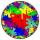Mark three points E, F and G in the plane not lie on one line. a) Draw a line segment FG b) Construct halfline (ray) EG c) Draw a line EF
4. Straight lines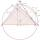Draw two lines c, d so that c || d. On line c mark points A, B, from point A start perpendicular to line c, from point B perpendicular to line c.
5. The sum graphically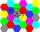Draw a graphically sum of the all sides of 4-gon ABCD.
6. Postal stamps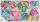Jano and Peter exchanged postal stamps. Jano gave Peter 32 stamps of the missile for 8 stamps with turtles. How good was Jano after this exchange (how many he has surplus in exchanged stamps)?
7. CollectionMajka gave from her collection of calendars Hanke 15 calendars, Julke 6 calendars and Petke 10 calendars. Still remains 77 calendars. How many calendars had Majka in her collection at the beginning?
8. Line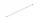How many parts of line divide 5 (different) points that lie on it?
9. Book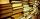Jitka read on holidays book that has 180 pages. In the first week read 45 pages. In the second week she read 15 pages more than the first week. How many pages left to read it yet?
10. Hotel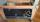The rooms in the mountain hotel are double and triple. Double rooms are 25 and triple are 17 more. How many rooms are there in this hotel?
11. Plane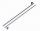On how many parts divide plane 6 parallels?
12. Bus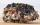On the 4-th stop take on 56 and take off 38 passengers. How many were added (write as positive number) or shrunk (write as negative number) the count of passengers?
13. Baking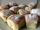There are 28 bunches, and son ate 1/2, dad ate four bunches. How many of them remain on the baking dishes?
14. Computer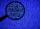A line of print on a computer contains 64 characters (letters, spacers or other chars). Find how many characters there are in 7 lines.
15. Mom and dad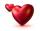Mom wants talk with dad 3 hours. But dad wants of 2 times more hours than mom ... How many hours wants dad talk with mom? ...
16. FedorFedor stood in the small pool. Above the surface was a part of his body that was twice as long as part of his body underwater. What height does Fedor have, if his body part below the surface is 5 dm long?
17. Fridays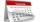Friday 13th is in 4 days. What day is today and what day it is?